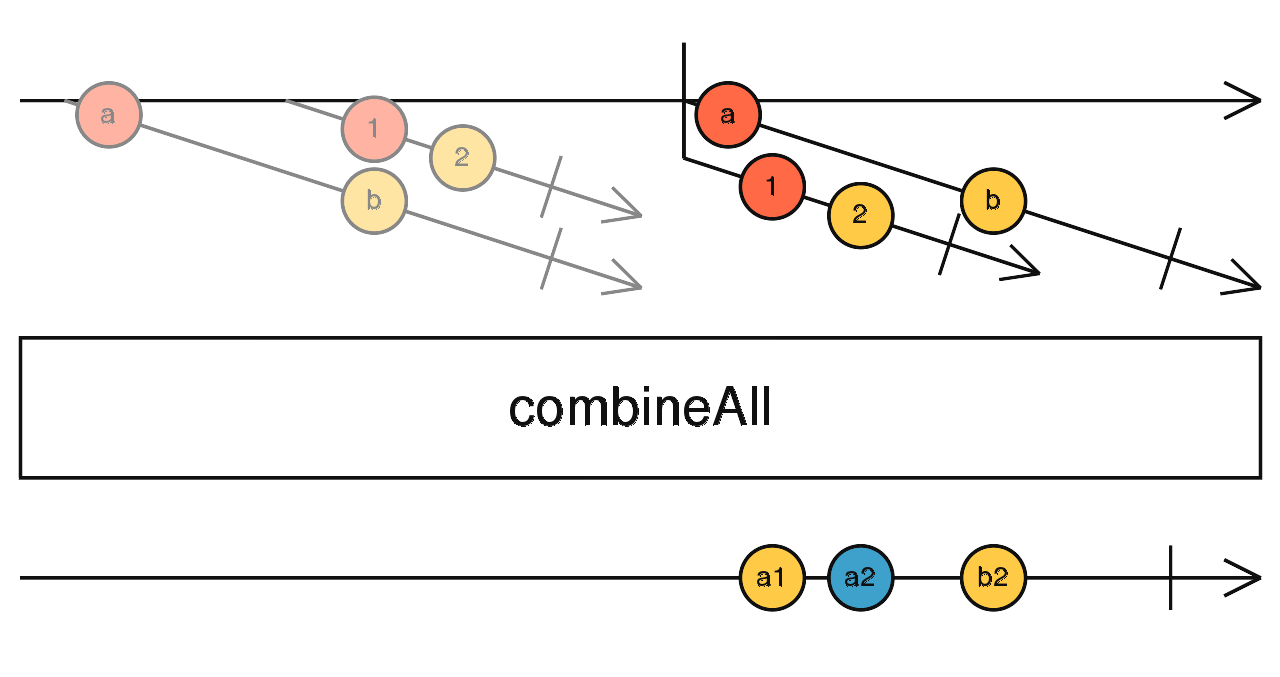/RxJS

# combineAll

`function` `stable`

Flattens an Observable-of-Observables by applying `combineLatest` when the Observable-of-Observables completes.

### `combineAll<T, R>(project?: (...values: Array<any>) => R): OperatorFunction<T, R>`

#### Parameters

 project Optional. Default is `undefined`. Type: `(...values: Array) => R`.

#### Returns

`OperatorFunction<T, R>`:

## Description`combineAll` takes an Observable of Observables, and collects all Observables from it. Once the outer Observable completes, it subscribes to all collected Observables and combines their values using the `combineLatest` strategy, such that:

• Every time an inner Observable emits, the output Observable emits
• When the returned observable emits, it emits all of the latest values by:

• If a `project` function is provided, it is called with each recent value from each inner Observable in whatever order they arrived, and the result of the `project` function is what is emitted by the output Observable.
• If there is no `project` function, an array of all the most recent values is emitted by the output Observable.

## Examples

#### Map two click events to a finite interval Observable, then apply `combineAll`

```import { fromEvent, interval } from 'rxjs';
import { map, combineAll, take } from 'rxjs/operators';

const clicks = fromEvent(document, 'click');
const higherOrder = clicks.pipe(
map(ev =>
interval(Math.random() * 2000).pipe(take(3))
),
take(2)
);
const result = higherOrder.pipe(
combineAll()
);

result.subscribe(x => console.log(x));```

### `combineAll(): OperatorFunction<ObservableInput<T>, T[]>`

#### Parameters

There are no parameters.

#### Returns

`OperatorFunction<ObservableInput<T>, T[]>`

### `combineAll(): OperatorFunction<any, T[]>`

#### Parameters

There are no parameters.

#### Returns

`OperatorFunction<any, T[]>`

### `combineAll(project: (...values: T[]) => R): OperatorFunction<ObservableInput<T>, R>`

#### Parameters

 project Type: `(...values: T[]) => R`.

#### Returns

`OperatorFunction<ObservableInput<T>, R>`

### `combineAll(project: (...values: Array<any>) => R): OperatorFunction<any, R>`

#### Parameters

 project Type: `(...values: Array) => R`.

#### Returns

`OperatorFunction<any, R>`